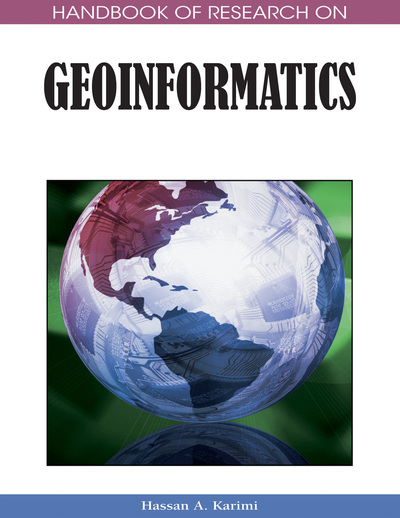# Artificial Neural Networks

Xiaojun Yang (Florida State University, USA)
DOI: 10.4018/978-1-59140-995-3.ch016

## Abstract

Artificial neural networks are increasingly being used to model complex, nonlinear phenomena. The purpose of this chapter is to review the fundamentals of artificial neural networks and their major applications in geoinformatics. It begins with a discussion on the basic structure of artificial neural networks with the focus on the multilayer perceptron networks given their robustness and popularity. This is followed by a review on the major applications of artificial neural networks in geoinformatics, including pattern recognition and image classification, hydrological modeling, and urban growth prediction. Finally, several areas are identified for further research in order to improve the success of artificial neural networks for problem solving in geoinformatics.
Chapter Preview
Top

## Background

The basic structure of an artificial neural network involves a network of many interconnected neurons. These neurons are very simple processing elements that individually handle pieces of a big problem. A neuron computes an output using an activation function that considers the weighted sum of all its inputs. These activation functions can have many different types but the logistic sigmoid function is quite common:

where f(x) is the output of a neuron and x represents the weighted sum of inputs to a neuron. As suggested from Equation 1, the principles of computation at the neuron level are quite simple, and the power of neural computation relies upon the use of distributed, adaptive and nonlinear computing. The distributed computing environment is realized through the massive interconnected neurons that share the load of the overall processing task. The adaptive property is embedded with the network by adjusting the weights that interconnect the neurons during the training phase. The use of an activation function in each neuron introduces the nonlinear behavior to the network.

There are many different types of neural networks, but most can fall into one of the five major paradigms listed in Table 1. Each paradigm has advantages and disadvantages depending upon specific applications. A detailed discussion about these paradigms can be found elsewhere (e.g., Bishop, 1995; Rojas, 1996; Haykin, 1999; and Principe et al., 2000). This article will concentrate upon multilayer perceptron networks due to their technological robustness and popularity (Bishop, 1995).

Table 1.
Classification of artificial neural networks (Source: Haykin, 1999)
 No. Type Example Brief description 1 Feed-forward neural network Multi-layer perceptron It consists of multiple layers of processing units that are usually interconnected in a feed-forward way Radial basis functions As powerful interpolation techniques, they are used to replace the sigmoidal hidden layer transfer function in multi-layer perceptrons Kohonen self-organizing networks They use a form of unsupervised learning method to map points in an input space to coordinate in an output space. 2 Recurrent network Simple recurrent networks Contrary to feed-forward networks, recurrent neural networks use bi-directional data flow and propagate data from later processing stages to earlier stages Hopfield network 3 Stochastic neural networks Boltzmann machine They introduce random variations, often viewed as a form of statistical sampling, into the networks 4 Modular neural networks Committee of machine They use several small networks that cooperate or compete to solve problems. 5 Other types Dynamic neural networks They not only deal with nonlinear multivariate behavior, but also include learning of time-dependent behavior. Cascading neural networks They begin their training without any hidden neurons. When the output error reaches a predefined error threshold, the networks add a new hidden neuron. Neuro-fuzzy networks They are a fuzzy inference system in the body which introduces the processes such as fuzzification, inference, aggregation and defuzzification into a neural network.

## Key Terms in this Chapter

Pruning Algorithm: A training algorithm that optimizes the number of hidden layer neurons by removing or disabling unnecessary weights or neurons from a large network that is initially constructed to capture the input-output relationship.

Feed-Forward: A network in which all the connections between neurons flow in one direction from an input layer, through hidden layers, to an output layer.

Architecture: The structure of a neural network including the number and connectivity of neurons. A network generally consists of an input layer, one or more hidden layers, and an output layer.

Neuron: The basic building block of a neural network. A neuron sums the weighed inputs, processes them using an activation function, and produces an output response.

Multiplayer Perceptron: The most popular network which consists of multiple layers of interconnected processing units in a feed-forward way.

Error Space: The n-dimensional surface in which weights in a networks are adjusted by the back-propagation algorithm to minimize model error.

Training/Learning: The processing by which the connection weights are adjusted until the network is optimal.

Back-Propagation: The training algorithm for the feed-forward, multi-layer perceptron networks which works by propagating errors back through a network and adjusting weights in the direction opposite to the largest local gradient.

## Complete Chapter List

Search this Book:
Reset